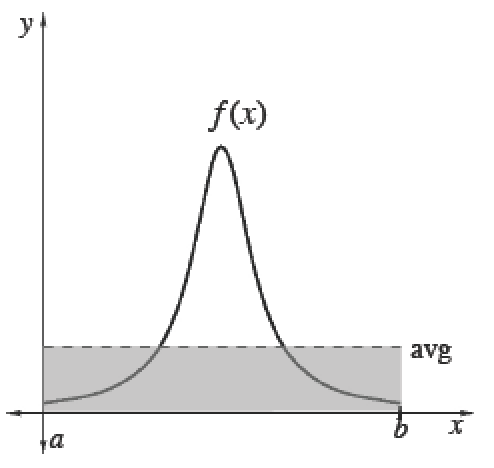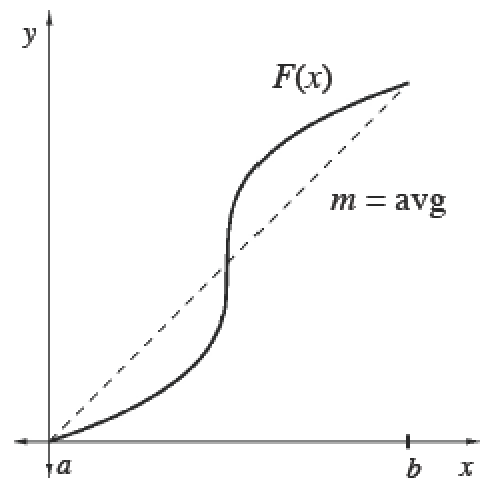### Home > APCALC > Chapter 8 > Lesson 8.2.1 > Problem8-74

8-74.

Multiple Choice: The average value of $f(x)=\ln(x^2)$ on the interval $1\le x\le e$ is closest to:

1. $0.50$

1. $0.65$

1. $0.75$

1. $0.85$

1. $1.00$

You are given $f(x)$ and you want to find the average (mean) value of $f(x)$. Will you integrate and divide, or will you find the slope of the secant line?

Average (Mean) Values

 To calculate the mean (average) value of a finite set of items, add up the values of items and divide by the number of items.Integrals help us add over a continuous interval. Therefore, for any continuous function $f$ :$\frac{\int_a^bf(x)dx}{b-a}=$ mean value of $f$ over $[a, b]$Since $\int _ { a } ^ { b } f ( x ) d x$, we can also calculate the average value of any function $f$ using its antiderivative $F$. Its average slope gives the average rate of change of $F$, which is the same as the average value of $f.$$\frac{\int_a^bf(x)dx}{b-a}=\frac { F ( b ) - F ( a ) } { b - a }=$  mean rate of change of $F ′$ over $[a, b]$